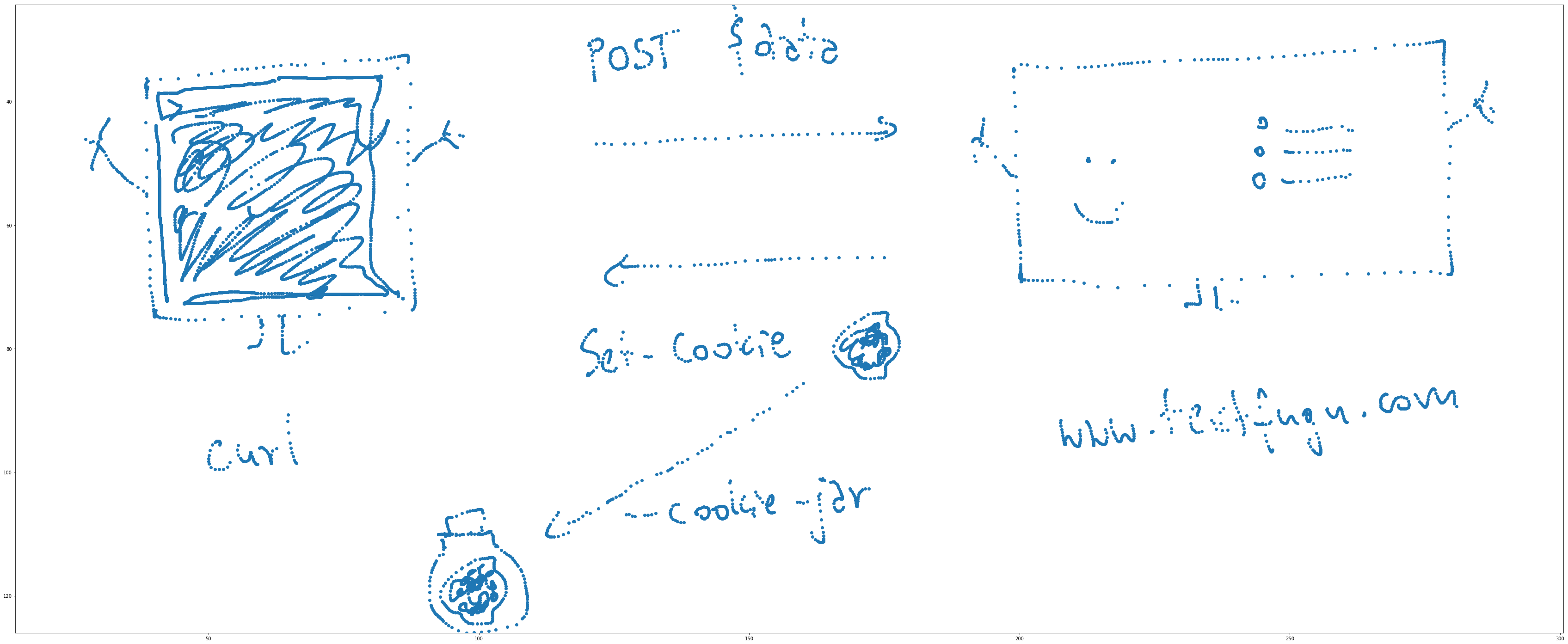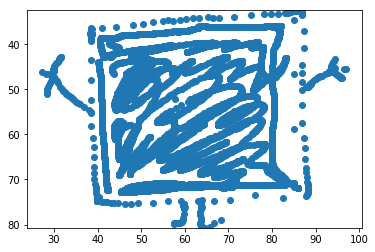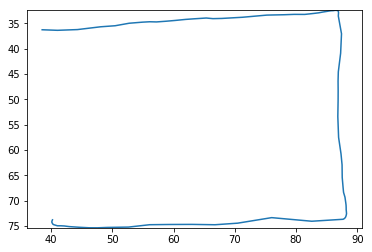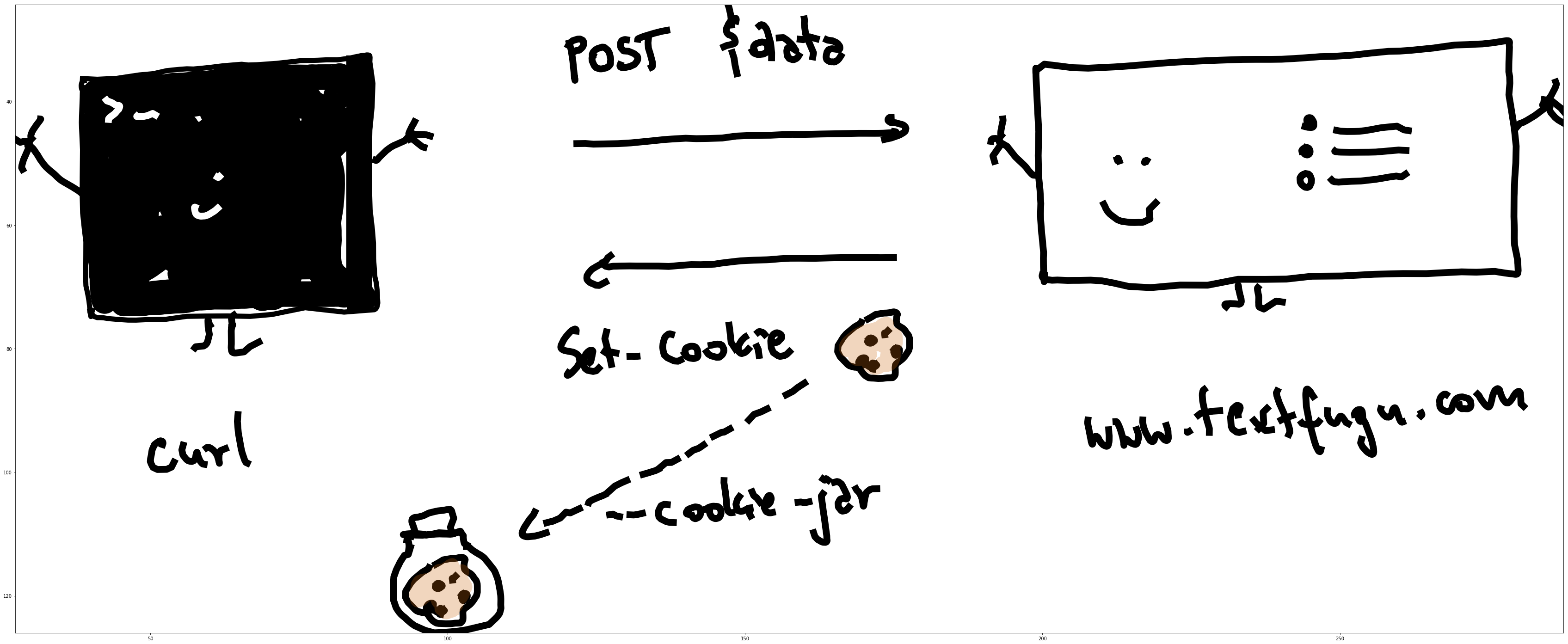I use Notability a lot to take notes while learning and during meetings. It’s fast and comfortable, allowing me to jot down my ideas on the fly. One caveat of taking notes in this way is that they’re not easily exportable in an editable format, so I can’t reuse them in other contexts (e.g. when creating diagrams to explain ideas).

I poked around and found a blog post by Julia Evans on reverse engineering the Notability format, which was a great starting point. My use case is a bit different, since I didn’t want to put my notes in the format - I wanted to get them out of it. It also seems that Notability has changed formats since then, but the initial ideas turned very helpful.

### Getting the data

I grabbed a note on curl and unzipped the file. I tried to use Julia’s code directly, but quickly realized the data is in a slightly different format. To decode it, I needed to use `biplist`, for binary plist files.

``````import biplist

note = './Blog_post/Session.plist'

try:
except e:
print('Not a readable plist: ', e)
``````

I then wanted to see what’s in the data and poke around a bit to see what I’m working with.

I needed to retrieve:

• data points for each point in the drawing
• some information on connecting the points
• some information on figuring out where a shape/curve ends
• colors and for the curves

I listed out the keys and decided `\$objects` looks promising. I followed with a highly scientific method of going through the keys of `\$objects` one by one to find something useful. An element that looked promising had keys `curvespoints` (sounds like the coordinates of the points) and `curvescolors` (might be the colors) in it, so it looked like I hit the spot.

``````print(plist.keys())
> dict_keys(['\$version', '\$objects', '\$archiver', '\$top'])
print(plist['\$objects'].keys())
> dict_keys(['eventTokens', 'curvesnumpoints', 'numfractionalwidths', 'curveswidth', 'curvesfractionalwidths', '\$class', 'curvescolors', 'curvespoints', 'numpoints', 'numcurves'])
``````

### Drawing initial data

I grabbed the points and unpacked them with `struct`. To make sure I have the right data, I plotted them.

``````import struct
import matplotlib.pyplot as plt

# directly using Julia's method with changing the result to int first
# also had to add some changes to process other formats than float (for colors etc.)
def unpack_struct(string, fmt, size):
return struct.unpack('{num}{format}'.format(num=int(len(string)/size), format=fmt), string)

drawings = plist['\$objects']
points = unpack_struct(drawings['curvespoints'], 'f', 4)

# let's see a scatterplot of the points
xs = points[0::2]
ys = points[1::2]

plt.figure(figsize=(60,25))
plt.ylim(max(ys), min(ys))
plt.scatter(xs, ys)
``````Yay! This looks like my drawing.

Now I just need to connect the curves, but how do I know when a shape ends? Maybe some value goes to 0? Let’s check the x values for the points.

``````[x for x in xs if x <= 0]
> []
``````

Hmm, it doesn’t seem like it. Let’s draw a shape by manually adjusting the range and see where it ends.

``````# after a few manual adjustments, this seems to be the shape
xs2 = xs[0:5036]
ys2 = ys[0:5036]

plt.ylim(max(ys2), min(ys2))
plt.scatter(xs2, ys2)

# let's like at what's next to the end of the shape
x0 = xs[5035:5040]
y0 = ys[5035:5040]

print(x0, y0)
> (64.0300064086914, 121.70161437988281, 123.13665771484375, 124.48757934570312, 126.95639038085938) (74.58029174804688, 46.82347106933594, 46.80168151855469, 46.90596008300781, 46.85621643066406)
``````Okay, let’s look at some other keys in `\$objects`. The `curvesnumpoints` seems like it can have some information on the number of points in each curve.

``````num_points = unpack_struct(drawings['curvesnumpoints'], 'i', 4)
num_points[0:10]
> (40, 85, 7, 4, 4, 16, 430, 1066, 379, 2713)
``````

Let’s draw a curve! It looks like the second curve starts at 40 and ends at 125 (40 + 85).

``````x1 = xs[40:125]
y1 = ys[40:125]

plt.ylim(max(y1), min(y1))
plt.plot(x1, y1)
``````Awesome! Looking at the keys in `\$objects`, the useful ones seem to be:

• `curvespoints` - the coordinates for each point
• `curvesnumpoints` - the lengths for curves (described as the number of points in a curve)
• `curveswidth` - the widths of curves
• `curvescolors` - the colors of curves, in rgba

With all that, I should be able to recreate the drawing.

### Putting it all together

To recreate the original drawing, for every element in `curvespoints` I need to:

• take the number of points in `curvesnumpoints`
• draw a line through them with the color specified in `curvescolors` and a width from `cruveswidth`
``````plt.figure(figsize=(60,25))
plt.ylim(max(ys), min(ys))
plt.xlim(min(xs), max(xs))

current_x = 0
current_y = 0

points = unpack_struct(drawings['curvespoints'], 'f', 4)
num_points = unpack_struct(drawings['curvesnumpoints'], 'i', 4)
widths = unpack_struct(drawings['curveswidth'], 'f', 4)
colors = unpack_struct(drawings['curvescolors'], 'B', 1)

# matplotlib uses fractions, so let's rerange colors
colors_reranged = [x / 255 for x in colors]
colors_list = [colors_reranged[i:i+4] for i in range(0, len(colors), 4)]

type(list(num_points))
for i, curve in enumerate(num_points):
max_x = current_x + curve
max_y = current_y + curve
plt.plot(xs[current_x:max_x], ys[current_y:max_y], color=colors_list[i], linewidth=10*widths[i])
current_x = max_x
current_y = max_y
``````I wrote a short script for retrieving drawings from Notability, you can grab it from this gist. It also has a copy of a Jupyter notebook I wrote this post in.